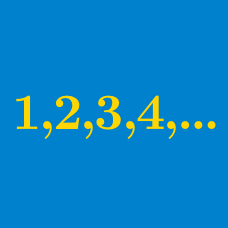Algebra

# Arithmetic Progressions: Level 3 Challenges

On the range of values from $[1, 1000]$, there exist a certain number of perfect squares. As you move from one perfect square to the next without skipping any, you will find that the distance between perfect squares increases. What is the average of all such distances in the given range of values?

$\left (1 + 3 + 5 + \ldots + p^\text{th} \right ) \\ + \\ \left (1 + 3 + 5 + \ldots + q^\text{th} \right ) \\ = \\ \left (1 + 3 + 5 + \ldots + r^\text{th} \right )$

Given the above equation for positive integers $p,q,r$ with $p^\text{th}$ term greater than 39.

What is the smallest possible value of the expression below?

$\large p^\text{th} \ \text{term } + q^\text{th} \ \text{term } + r^\text{th} \ \text{term }$

$\dfrac{a(q-r)}{p} + \dfrac{b(r-p)}{q} + \dfrac{c(p-q)}{r}$

In an arithmetic progression, the sum of the first $p, q, r$ terms are $a, b, c$ respectively. Compute the expression above.

Consider an arithmetic progression with 2 and 101 as its first term and last term respectively. If the sum of the first 5 terms of this arithmetic progression is 40, find the sum of the last 5 terms of this progression.

$\begin{array}{llllll} A_1 : & 2, & 9, & 16, \ldots , & 2 + (1000-1) \times 7 \\ A_2: & 3, & 12 , & 21, \ldots, & 3 + (1000-1) \times 9? \\ \end{array}$

How many integers appear in both of the following arithmetic progressions above?

Details and assumptions

Since 2 appears in $A_1$ but not in $A_2$, it does not appear in both of the arithmetic progressions.

$\large \frac ab \ , \ ab \ , \ a -b \ , \ a+b$

Above shows real numbers that belong to an arithmetic progression in order. Find the next term of this sequence.

×